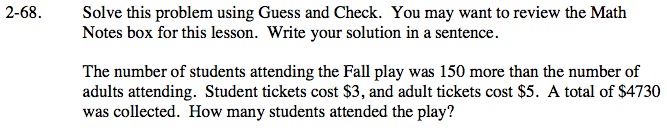### Home > CAAC > Chapter 2 > Lesson 2.1.7 > Problem2-68

2-68.

Solve this problem using Guess and Check. You may want to review the Math Notes box for this lesson. Write your solution in a sentence. Homework Help ✎

The number of students attending the Fall play was 150 more than the number of adults attending. Student tickets cost $3, and adult tickets cost$5. A total of $4730 was collected. How many students attended the play?Start by making a guess for the number of adults, and use that to calculate the number of students. How much was collected in adult money, and how much was collected in student money? Find the total and see if it is$4730.

If the total is not \$4730, try again.